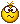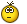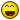# How does this work? Confused.com!

#### smokeysmoo

##### Legendary Member
LINKY•MossCommuter

#### vernon

##### Harder than Ronnie Pickering
LINKYI could tell you but then I'd have to kill you.

•smokeysmoo

#### vernon

##### Harder than Ronnie Pickering
Clue:

It can be found in: Synergetics, Explorations in the Geometry of Thinking. on page 765

#### Jefferson Meriwether

##### Veteran
I haven't got a scooby how that works. I tried it with a 6 digit number and it still guessed right.

#### vernon

##### Harder than Ronnie Pickering
I haven't got a scooby how that works. I tried it with a 6 digit number and it still guessed right.
It will work with any number of digits......

#### Red Light

##### Guest
Shamelessly stolen from elsewhere on the Interwebby:

The Think Clear Puzzle

This little puzzle stems from properties of our old friend the digit 9. The
difference between any number and the jumbling of its digits produces a multiple of 9.
Since most of us use the decimal system to communicate numbers, we can express any number as a sum of the digits times powers of 10. Symbolically, the number N can be expressed as N = 10^n*A1 + 10^(n-1)*A2 + ...+An where A1,A2,...An are the n digits of the number. For example if we choose the number 3141 in powers of 10 it becomes 10^3*3 + 10^2*1 + 10*4 + 1 or 1000*3 + 100*2 + 10*4 +1 = 3000+100 + 40 +1 = 3141.
Let us subtract symbolically a 4 digit number and its jumble. Let N = 1000A1 + 100A2 + 10A3 + A4 Now we know there are 24 possible ways to jumble A1 to A4. Eg., A4A3A2A1, A3A4A2A1 etc. Let J = 1000A2 + 100A1 + 10A4 + A3. Now if we take N-J we are free to subtract any term in J from any term from N (Commutative law of addition) for all terms and add them up. In our example, N-J = 1000A1-100A1 +100A2-1000A2 +10A3-A3 + A4-10A4. This factors to N-J = 900A1 – 900A2 + 9A3 - 9A4 = a multiple of 9. Notice that no matter how we arrange the A's in the jumble there is always a corresponding A in N such that when we subtract two terms, we will have a multiple of 9 for each term of the difference-pair. Therefore, the difference between any number and its jumble (with or without regard to sign) is a multiple of 9.
The following Theorem will enable us to complete the puzzle.
Digital Root Theorem:
The digital root of an integer x is 9 if 9 divides x or the remainder of x/9 if 9 does not divide x.
The digital root of a number is the repeated summing of the digits until you get a single digit. For example, the Digital root of 1234567 = 1+2+3+4+5+6+7 = 28. 2+8=10.1+0 = 1.
Using the theorem, 1234567/9 = 137174*9 + 1. So the remainder is 1 as we would expect. Now if we have a number that is a multiple of 9 say, 12345678 then we get a remainder 0 which implies 9 is a divisor as is the case in difference the puzzle. Then taking away a digit d will leave a new sum of 9 - d. So if we circle the 8 in 12345678 we will have a new digital root of 1. So 9 – d is 1 and d is 8.
The Puzzle cleverly says to pick non-zero so to hide the 9 property. You will always get 9 if you pick 0 because 0 lets the digital root stay at 9 and 9 - 9 = 0.
I will summarize:
Using Excel, you can dazzle your friends at work without the boss catching you on the web of course.
Rows 3 and 6 are the formulas 1,2,4 are input. 6 is also the answer.
A B
1 Number =>
2 Jumble =>
3 Difference =ABS(A1-B1) Take the positive number
4 Picked digit=>
5
6 Digit picked =9 - MOD(B4,9) Justified by the digital root Theorem

So know you know#### vernon

##### Harder than Ronnie Pickering
So know you knowIt's known as 'Casting Out Nines' and is used as a quick check for errors in calculations.

#### Judderz

##### Well-Known Member
He actually gets mine wrong, keeps saying I picked a '6', but I picked a '9'

#### smokeysmoo

##### Legendary Member
He actually gets mine wrong, keeps saying I picked a '6', but I picked a '9'
I know why that is.

6 = Neil

9 = Phil#### Judderz

##### Well-Known Member
I know why that is.

6 = Neil

9 = PhilHaha...gonna call you Fido from now on then :P

•NotthatJasonKenny and smokeysmoo